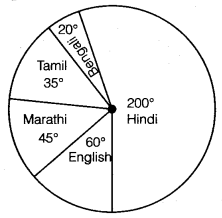# Class 8 Maths NCERT Solutions for Chapter – 5 Data Handling Ex – 5.2

## Data Handling

Question 1.
A survey was made to find the type of music that a certain group of young people liked in a city. Following pie chart shows the findings of this survey.

From this pie chart answer the following:
(i) If 20 people liked classical music, how many young people were surveyed?
(ii) Which type of music is liked by the maximum number of people?
(iii) If a cassette company were to make 1000 CD’s, how many of each type would they make?

Solution:
(i) Let x be the number of young people surveyed.
∴ 10% of x = 20
⇒$\frac { 10 }{ 100 }$ x x = 20
⇒ x = 20 x 10 = 200
Thus, number of young people surveyed = 200
(ii) Light music is liked by the maximum number of people.
(iii) Number of CD’s in respect of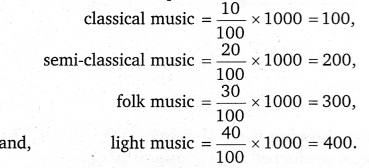Question 2.
A group of 360 people were asked to vote for their favourite season from the three seasons rainy, winter and summer.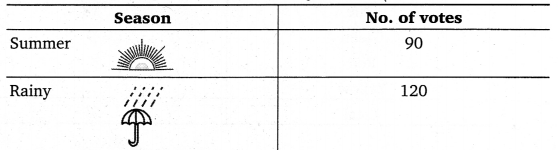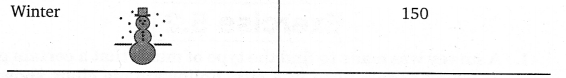(i) Which season got the most votes?
(ii) Find the central angle of each sector.
(iii) Draw a pie chart to show this information.

Solution:
(i) The winter season got the maximum votes.
(ii) The proportion of sectors (winter, summer and rainy seasons) are as

(iii)
Central angle for winter season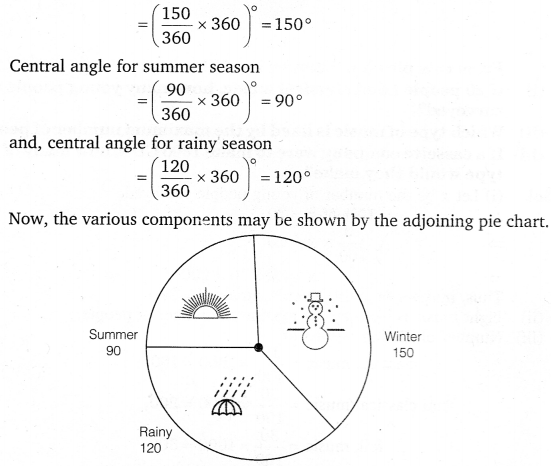Question 3.
Draw a pie chart showing the following information. The table shows the colours preferred by a group of people.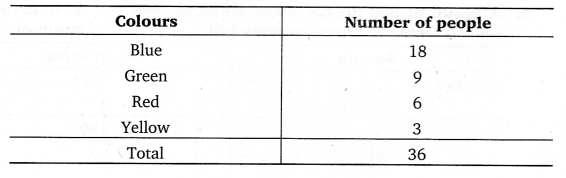Find the proportion of each sector. For example. Blue is$\frac { 18 }{ 36 } =\frac { 1 }{ 2 }$; Green is$\frac { 9 }{ 36 } =\frac { 1 }{ 4 }$ and so on.
Use this to find the corresponding angles.

Solution:
Central angle for the blue colour$=\left( \frac { 18 }{ 36 } \times 360 \right) ^{ \circ }=180^{ \circ }$,
Central angle for the green colour$=\left( \frac { 9 }{ 36 } \times 360 \right) ^{ \circ }=90^{ \circ }$,
Central angle for the red colour$=\left( \frac { 6 }{ 36 } \times 360 \right) ^{ \circ }=60^{ \circ }$,
central angle for the yellow colour$=\left( \frac { 3 }{ 36 } \times 360 \right) ^{ \circ }=30^{ \circ }$,
Now, various components may be shown by the adjoining pie chart.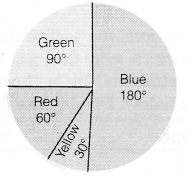Question 4.
The adjoining pie chart gives the marks scored in an examination by a student in Hindi, English, Mathematics, Social Science and Science. If the total marks obtained by the students were 540, answer the following questions.

(i)
In which subject did the student score 105 marks?
(ii) How many more marks were obtained by the student in Mathematics than in Hindi?
(iii) Examine whether the sum of the marks obtained in Social Science and Mathematics is more than that in Science and Hindi.

Solution:
(i) For 540 marks, the central angle = 360°
∴ For 1 mark, the central angle =$\left( \frac { 360 }{ 540 } \right) ^{ \circ }=\left( \frac { 2 }{ 3 } \right) ^{ \circ }$
∴ For 105 marks, the central angle =$\left( \frac { 2 }{ 3 } \times 105 \right) ^{ \circ }=70^{ \circ }$
Hence, from the given pie chart, the subject is Hindi.

(ii) Difference between the central angles made by the subject of Mathematics and Hindi = 90° – 70° = 20°.
For the central angle of 360°, marks obtained = 540
For the central angle of 1°, marks obtained$=\frac { 540 }{ 360 } =\frac { 3 }{ 2 }$
For the central angle of 20°, marks obtained$=\frac { 3 }{ 2 } \times 20=30$
Hence, 30 more marks were obtained by the student in Mathematics than Hindi.

(iii) Sum of the central angles made by Social Science and Mathematics = 65°+ 90° = 155°.
And, the sum of the central angles made by Science and Hindi = 80°+ 70° = 150°.
Since 155° >150°, therefore, the sum of the marks obtained in Social Science and Mathematics is more than that in Science and Hindi.

Question 5.
The number of students in a hostel, speaking different languages is given below. Display the data in a pie chart.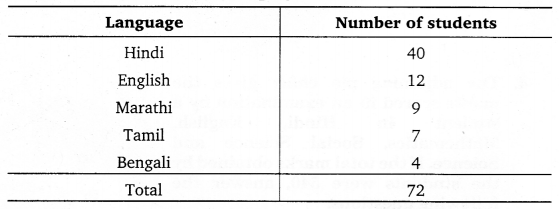Solution:
Let us find the central angle of each sector. Here the total scale = 72. We thus have the following table :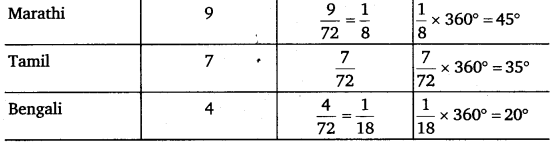Now, we make the pie chart as shown below :